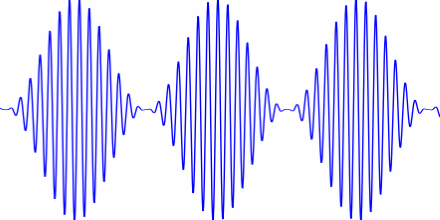# What is an asper?

Acoustic roughness is measured in aspers (from the Latin word for rough). An asper is the roughness of a 1 kHz tone, at 60 dB, 100% modulated at 70 Hz. That is, the signal

(1 + sin(140πt)) sin(2000πt)

where t is time in seconds.Here’s what that sounds like (if you play this at 60 dB, about the loudness of a typical conversation at one meter):

And here’s the Python code that made the file:

```
from scipy.io.wavfile import write
from numpy import arange, pi, sin, int16

def f(t, f_c, f_m):
# t    = time
# f_c  = carrier frequency
# f_m  = modulation frequency
return (1 + sin(2*pi*f_m*t))*sin(2*f_c*pi*t)

def to_integer(signal):
# Take samples in [-1, 1] and scale to 16-bit integers,
# values between -2^15 and 2^15 - 1.
return int16(signal*(2**15 - 1))

N = 48000 # samples per second
x = arange(3*N) # three seconds of audio

# 1 asper corresponds to a 1 kHz tone, 100% modulated at 70 Hz, at 60 dB
data = f(x/N, 1000, 70)
write("one_asper.wav", N, to_integer(data))
```